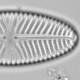## REDFIT’s rule of thumb

Because REDFIT tests many frequencies, some are likely to appear statistically significant just by chance — a classic multiple testing problem.  Schulz & Mudelsee (2002) “follow Thomson (1990) and select a false-alarm level of (1-1/n)*100%, where n is the number of data points in each WOSA segment.”

How good is this rule-of-thumb? This can be tested with simulated data, observing how often this critical false-alarm level is exceeded. With a data set of 200 observations and three WOSA segments, n in each segment is 100, so 1-1/n*100% is 99%, a significance level that REDFIT has conveniently already calculated. I’m going to test this rule-of-thumb with simulated data with different strengths of autocorrelation.

```ar1=seq(.1,.9,.1)
res<-sapply(ar1,function(p){
t1<-replicate(100,{
x<-data.frame(1:200, as.vector(arima.sim(list(ar=p), n=200)))
rdf<-redfit(x)     c(any(rdf\$redfit[,3]>rdf\$redfit[,10]),any(rdf\$redfit[,3]>rdf\$redfit[,14]))
})
rowMeans(t1)
})
x11(4,4)
par(mar=c(3,3,1,1), mgp=c(1.5,.5,0))
matplot(ar1,t(res), type="l", xlab="AR1", ylab="Fraction trials exceeding false alarm level")
```Proportion of trials that have a periodicities that exceeds the critical false alarm level for the Chi-sq test (black) and the Monte Carlo test (red).

It would appear that this rule-of-thumb is rather liberal; even with random data it will suggest that there are periodicities in many datasets. Even so, the rule-of-thumb is much better than naively interpreting any periodicities that exceed the 95% significance level as meaningful.

This rule-of-thumb only applies if the data are being examined in an exploratory fashion, it is not needed if someone is interested, a priori , in one periodicity only, for example 11 yr exactly. Here there is no multiple testing, so the 95% significance level from REDFIT is correct. If a band of periodicities is of interest, for example 9–13 years, multiple periodicities are being tested, so the 95% significance level will be liberal.

Frescura et al (2007) propose a Monte Carlo procedure for generating false alarm levels, that could be used when testing either the full spectrum or a narrow band of it. This procedure might be more useful that the rule-of-thumb.

I am becoming convinced that many of the papers that use REDFIT to describe solar periodicities in their data set are describing noise.1.Manfred Mudelsee says: